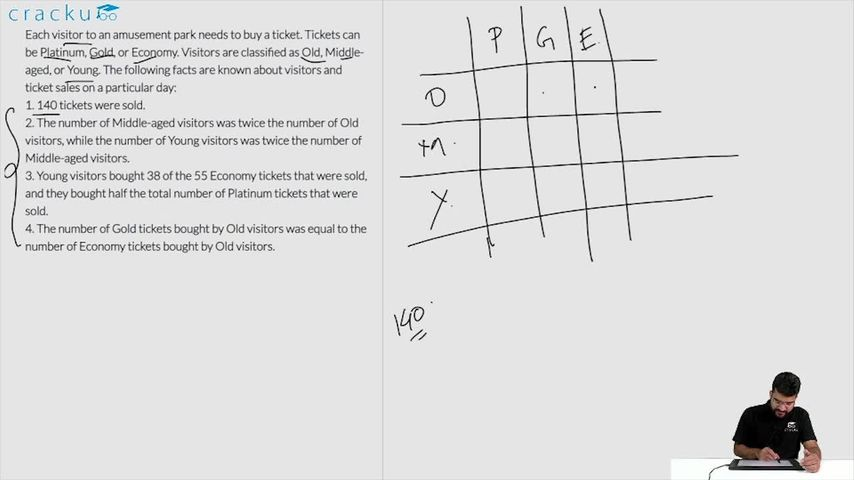### CAT 2018 Question Paper (Slot 2) Question 42

Instructions

Each visitor to an amusement park needs to buy a ticket. Tickets can be Platinum, Gold, or Economy. Visitors are classified as Old, Middle-aged, or Young. The following facts are known about visitors and ticket sales on a particular day:
1. 140 tickets were sold.
2. The number of Middle-aged visitors was twice the number of Old visitors, while the number of Young visitors was twice the number of Middle-aged visitors.
3. Young visitors bought 38 of the 55 Economy tickets that were sold, and they bought half the total number of Platinum tickets that were sold.
4. The number of Gold tickets bought by Old visitors was equal to the number of Economy tickets bought by Old visitors.

Question 42

# Which of the following statements MUST be FALSE?

Solution

Let 'x' be the the number of Old visitors. Then, the number of middle-aged visitors = 2x.

Also, the number of Young visitors = 2*2x = 4x

$$\Rightarrow$$ x+2x+4x = 140

$$\Rightarrow$$ x = 20

It is given that total of 55 Economy tickets were sold out.

It is given that Young visitors half the total number of Platinum tickets that were sold.

Let 'Y' be the number of Platinum tickets bought by the Young visitors.

Then,the number of Platinum tickets sold = 2Y.

Consequently, we can say that the number of Gold tickets sold = 140 - 55 - 2Y = 85 - 2Y.

Let us assume that 'Z' is the number of Economy tickets bought by the Old visitors. It is given that the number of Gold tickets bought by Old visitors was equal to the number of Economy tickets bought by Old visitors.

Let us check with the help of options.

Option (A): The numbers of Gold and Platinum tickets bought by Young visitors were equal.

Y = 42 - Y

$$\Rightarrow$$ Y = 21. Hence, this statement can be true.

Option (B): The numbers of Middle-aged and Young visitors buying Gold tickets were equal

43 - (Y+Z) = 42 - Y

$$\Rightarrow$$ Z = 1. Hence, this statement can be true.

Option (C): The numbers of Old and Middle-aged visitors buying Platinum tickets were equal

20 - 2Z = (Y+2Z) - 20

$$\Rightarrow$$ Y+4Z = 40. Hence, this statement can be true.

Option (D): The numbers of Old and Middle-aged visitors buying Economy tickets were equal

Z = 17 - Z

$$\Rightarrow$$ Z = 8.5. This is not possible as Z has to be an integer. Hence, we can say that this statement is false.

### View Video Solution